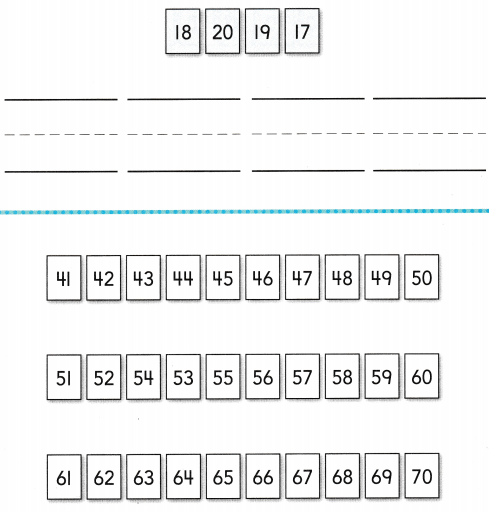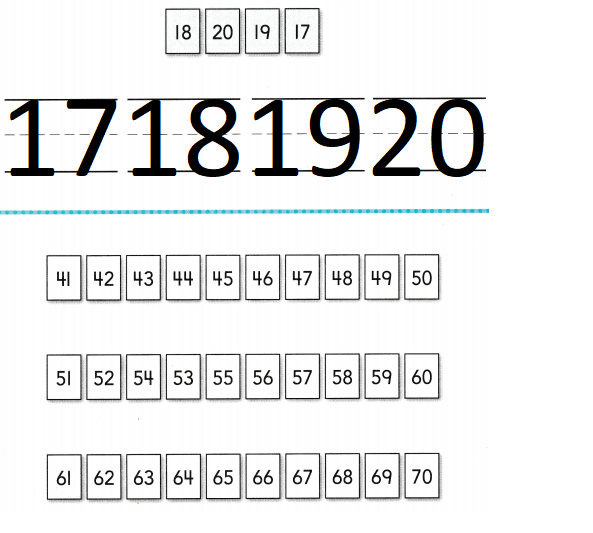# Texas Go Math Kindergarten Unit 3 Assessment Answer Key

Refer to our Texas Go Math Kindergarten Answer Key Pdf to score good marks in the exams. Test yourself by practicing the problems from Texas Go Math Kindergarten Unit 3 Assessment Answer Key.

## Texas Go Math Kindergarten Unit 3 Assessment Answer Key

Vocabulary

Question 1.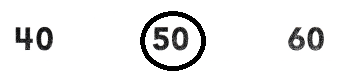Explanation:
Circled the number 50

Question 2.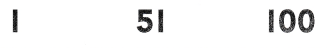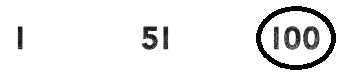Explanation:
Circled the number one hundred

Concepts and Skills

Question 3.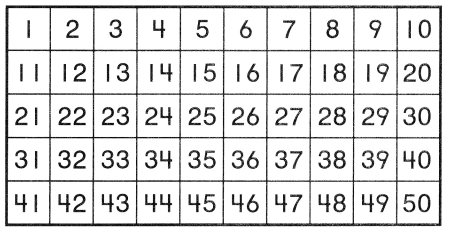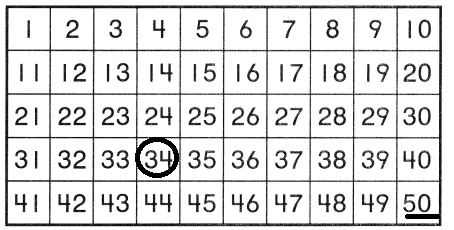Explanation:
Counted the numbers from 34 to 50

Question 4.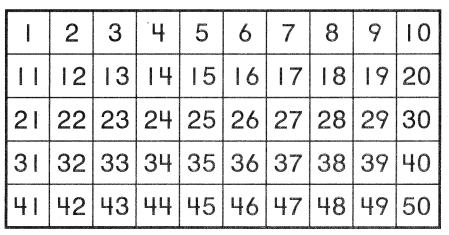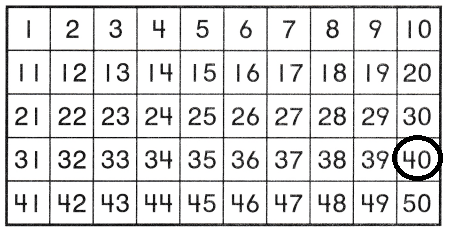Explanation:
The number is one more than 39 is 40
3 9 + 1 = 40
so, circled the number 40.

Directions
1. Circle the number fifty. 2. Circle the number one hundred. 3. Circle the number 34 Begin with 34 and count forward to 50. Underline the number 50. TEKS K.5 4. What number is one more than 39? Circle that number.
Begin with that number and count forward to 50. TEKS K.5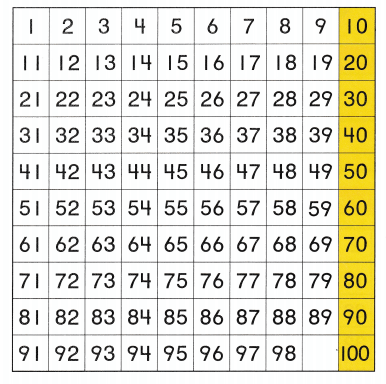Question 5.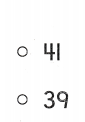Explanation:
one less than 40 is 39
40 – 1 = 39
so, marked the number 39.

Question 6.Explanation:
In the above 100 chart the missing number is 99
so, marked the number 99.

Question 7.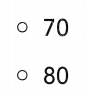Explanation:
The number that comes right after 60
is 70
It is count by 10’s

Directions
Fill in the circle for the correct answer choice. 5. Look at the hundred chart. What number is one less than 40? TEKS K.5 6. Look at the hundred chart. What number is missing? TEKS K.5 7. Look at the column highlighted in yellow on the hundred chart. When counting by tens, what number comes right after 60? TEKS K.5

Question 8.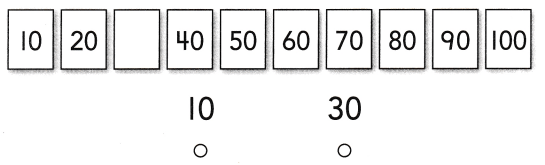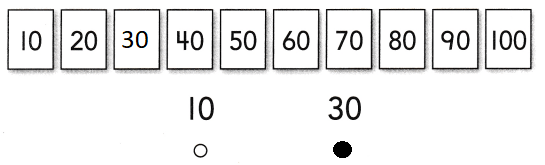Explanation:
In the above given table
the missing number is 30
so, marked the number.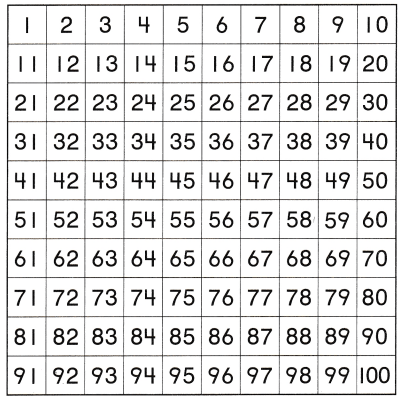Question 9.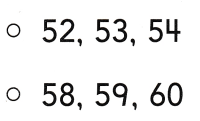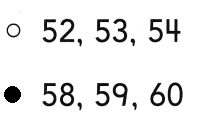Explanation:
three numbers that come after 57
are 58, 59 , 60.
so, marked those numbers

Question 10.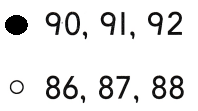Explanation:
three numbers that come after 89
are 90 , 91 , 92 ,
so, marked those numbers

Directions
Fill in the circle for the correct answer choice. 8. Count by tens. What is the missing number? TEKS K.5 9. Look at the hundred chart. Which three numbers come after 57? TEKS K.5 10. Look at the hundred chart. Which three numbers come after 89? TEKS K.5